## Compositions of Functions

When the input in a function is another function, the result is called a composite function. If

f ( x) = 3 x + 2 and g( x) = 4 x – 5

then f [ g( x)] is a composite function. The statement f [ g( x)] is read “ f of g of x” or “the composition of f with g.” f [ g( x)] can also be written as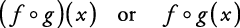The symbol between f and g is a small open circle. When replacing one function with another, be very careful to get the order correct because compositions of functions are not necessarily commutative (as you'll see).

##### Example 1

If f ( x) = 3 x + 2 and g( x) = 4 x – 5, find each of the following.

1. f [ g(4)]

2.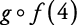3. f [ g( x)]

4.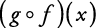1.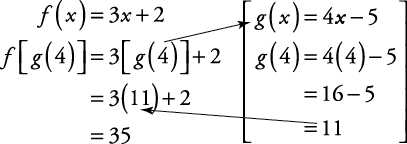2.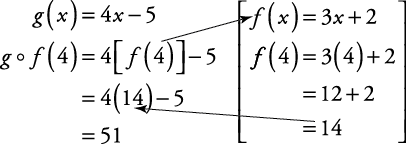3.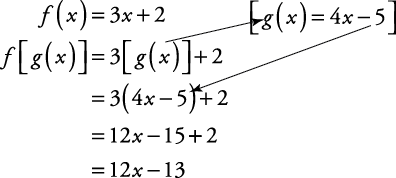4.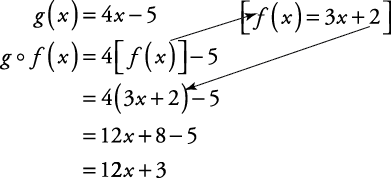From the previous example, you should be convinced that f [ g( x)] and g[ f ( x)] are not (necessarily) equal.

##### Example 2

If f( x) = 3 x 2 + 2 x + 1 and g( x) = 4 x – 5, find each of the following:

1. f [ g( x)]

2. g[ f ( x)]

1.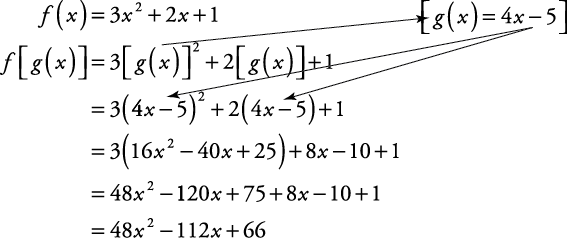2.Back to Top
A18ACD436D5A3997E3DA2573E3FD792A